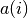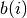# Silhouettes¶

Silhouettes is a method for validating clusters of data. Particularly, it provides a quantitative way to measure how well each item lies within its cluster as opposed to others. The Silhouette value of a data point is defined as:Here,is the average distance from the i-th point to other points within the same cluster. Letbe the average distance from the i-th point to the points in the k-th cluster. Thenis the minimum of allover all clusters that the i-th point is not assigned to.

Note that the value ofis not greater than one, and thatis close to one indicates that the i-th point lies well within its own cluster.

silhouettes(assignments, counts, dists)

Compute silhouette values for individual points w.r.t. a given clustering.

Parameters: assignments – the vector of assignments counts – the number of points falling in each cluster dists – the pairwise distance matrix It returns a vector of silhouette values for individual points. In practice, one may use the average of these silhouette values to assess given clustering results.
silhouettes(R, dists)

This method accepts a clustering result R (of a sub-type of ClusteringResult).

It is equivalent to silhouettes(assignments(R), counts(R), dists).